Author: Oscar Cronquist Article last updated on November 17, 2018

Question: How do I highlight dates that meet criteria using conditional formatting?

Highlight values in a column

Highlight values in a row

Highlight records

Sort values

### Highlight values in a column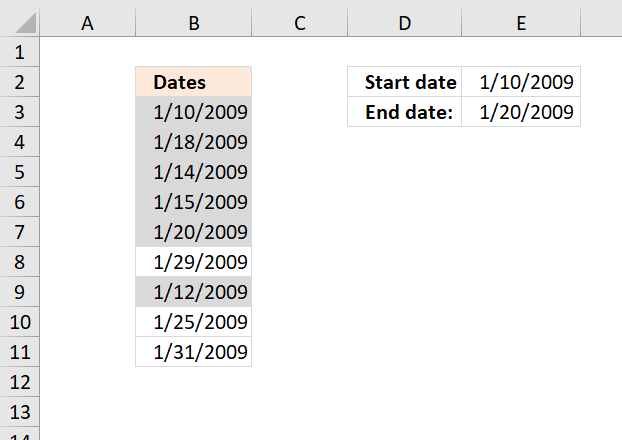### How to apply the conditional formatting formula

1. Select the range (B3:B11)
2. Press with left mouse button on "Home" tab on the ribbon
3. Press with left mouse button on "Conditional formatting"
4. Press with left mouse button on "New rule..."
5. Press with left mouse button on "Use a formula to determine which cells to format"
6. Press with left mouse button on "Format values where this formula is true" window.
7. Type
=(B3<=\$E\$3)*(B3>=\$E\$2)
8. Press with left mouse button on Format button
9. Press with left mouse button on "Fill" tab
10. Select a color
11. Press with left mouse button on OK!
12. Press with left mouse button on OK!

### Explaining CF formula

B3<=\$E\$2

becomes

39823<=39833

and returns TRUE

#### Step 2 - Check if current cell date is larger than or equal to start date

B3>=\$E\$2

becomes

39823>=39823

and returns TRUE.

#### Step 3 - Multiply boolean values

Both boolean values must be TRUE in order to highlight the cell.

 Boolean Boolean Multiply Add FALSE FALSE 0  (zero) 0 (zero) FALSE TRUE 0  (zero) 1 TRUE TRUE 1 2

The parentheses make sure that the order of calculation is correct.

(B3<=\$E\$3)*(B3>=\$E\$2)

becomes

TRUE*TRUE

and returns 1 which is equivalent to TRUE. Cell B3 is highlighted.

### Highlight values in a row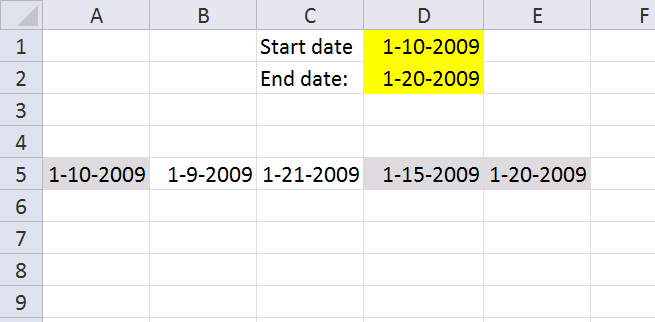Conditional formatting formula in cell range A5:E5:

=(A5<=\$D\$2)*(A5>=\$D\$1)

This formula is the same as the above (not the cell references though), the difference is that it is applied to a horizontal cell range.

### Highlight records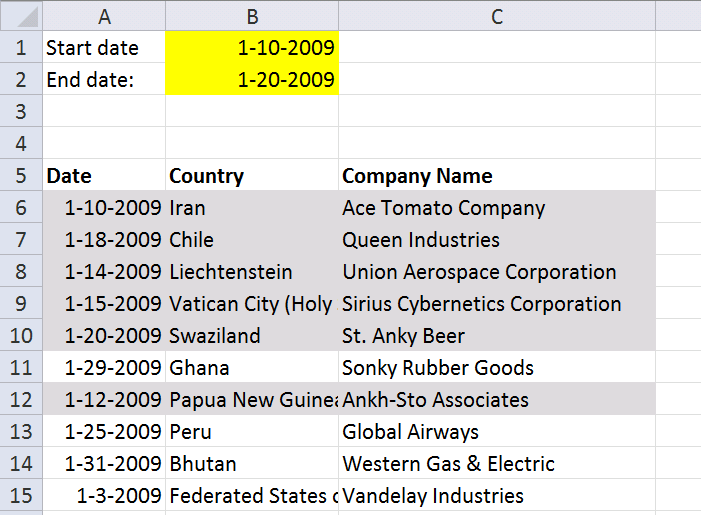Conditional formatting formula in cell range A6:C15:

=(\$A6<=\$B\$2)*(\$A6>=\$B\$1)

The cell references are "locked" to column A, that is why there is a dollar sign in front of \$A6. That makes the entire row highlighted if the date cell is in the date range.

### Sort values

You can quickly sort highlighted records to the top of the list.

1. Select cell range A6:C15
2. Press with right mouse button on on cell range
3. Press with left mouse button on "sort"
4. Press with left mouse button on "Put selected cell color on top"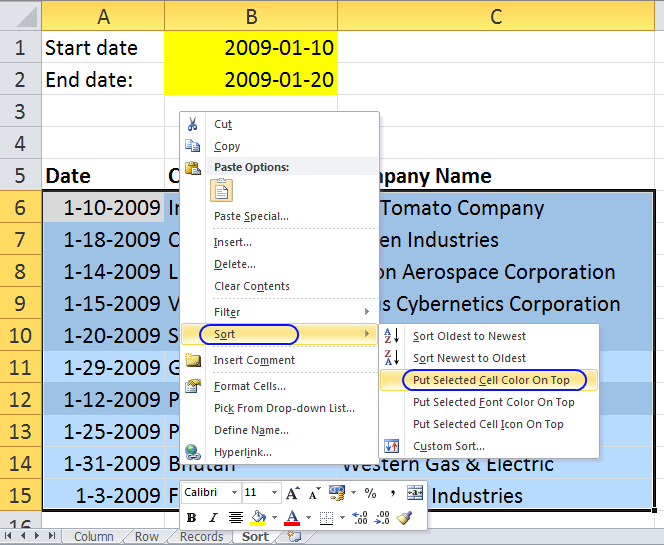All highlighted records are on top.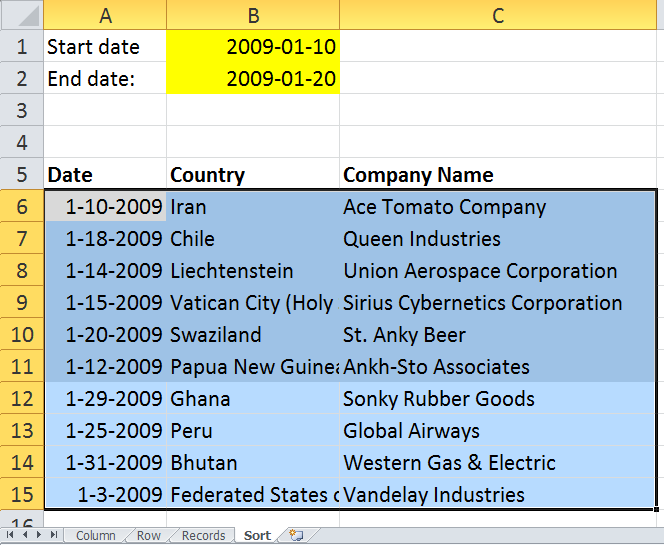Get excel *.xls file

highlight-dates-in-range-using-conditional-formatting2.xls

(Excel 97-2003 Workbook *.xls)